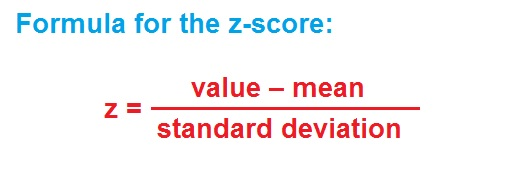﻿ How to find and interpret the z-score - MathBootCamps

# How to find and interpret the z-score

The z-score is a way of counting the number of standard deviations between a given data value and the mean of the data set. In this lesson, we will look at the formula for the z-score, how to calculate it, and a little more closely at this idea of counting standard deviations.

## The formula

To calculate the z-score, you first find the distance from the mean, and then divide by the standard deviation.If we label the value $$x$$, we could write the formula as:## Calculating and interpreting the z-score

Let’s look at an example to see how to use this formula.

### Example

The mean score on a standardized test was 508 with a standard deviation of 42. One test-taker’s score was 590. Find and interpret the z-score for this score.

From the example, we have the following information:

• The mean is: $$\mu = 508$$
• The standard deviation is: $$\sigma = 42$$

Therefore, the z-score is:

\begin{align}z &= \dfrac{x-\mu}{\sigma}\\ &= \dfrac{590 – 508}{42}\\ &= \dfrac{82}{42}\\ &\approx \boxed{1.95}\end{align}

When it comes to interpreting, you should note that by subtracting the mean from a data value, we will get a negative if it is smaller than the mean and a positive if it is larger. So, the sign gives the “direction” away from the mean. By dividing this difference by the standard deviation, we are putting this distance between the mean and the data value in terms of a number of standard deviations. Therefore, we can say:

“The test-score of 590 is about 1.95 standard deviations above the mean.”

Interpreting the z-score:
For a given data value, the z-score gives the number of standard deviations above (positive) or below (negative) the mean.

As you saw above, the value and the sign of the z-score gives you information about the location of the data value. Specifically:

• A positive z-score means the data value is larger than the mean.

If a data value has a z-score of 2, that tells us that this data value is 2 standard deviations larger than the mean.

• A negative z-score means the data value is smaller than the mean.

If a data value has a z-score of –3.1, then this data value is 3.1 standard deviations smaller than the mean.

• A z-score of zero means that the data value equals the mean.

For example, consider a data set with a mean of 50 and a standard deviation of 2. If a data value also is 50, then the z-score is:

\begin{align}z &= \dfrac{x-\mu}{\sigma}\\ &= \dfrac{50 – 50}{2}\\ &= \dfrac{0}{2}\\ &= \boxed{0}\end{align}

This will be the case anytime the mean and the data value are the same.

## Conclusion

In your study of statistics, you will come across the z-score in a wide variety of settings. For this reason, it is important to make sure you thoroughly understand the ideas discussed here. The best way to do this is to practice the calculation so that it simply becomes second nature! This will help make future topics much easier to understand.